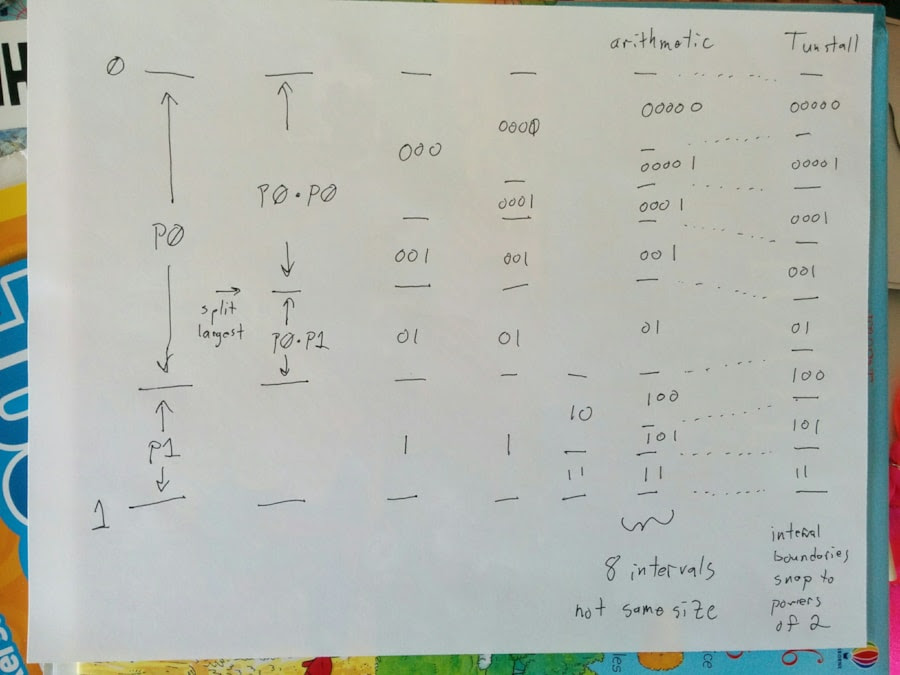# cbloom rants

## 4/15/2017

### Tunstall in an arithmetic way

You can think of the Tunstall dictionary build in an arithmetic codery way.

Your dictionary is like the probability interval. You start with a full interval [0,1]. You put in all the single-character words, with P(c) for each char, so that all sums to one. You iteratively split the largest interval, and subdivide the range.

```
In binary the "split" operation is :

W -> W0 , W1

P(W) = P(W)*P(0) + P(W)*P(1)

In N-ary the split is :

W -> Wa , W[b+]

P(W) = P(W)*P(a) + P(w)*Ptail(b)

W[b+] means just the word W, but in the state "b+" (aka state "1"), following sym must be >= b
(P(w) here means P_naive(w), just the char probability product)

W[b+] -> Wb , W[c+]

P(w)*Ptail(b) = P(w)*P(b) + P(w)*Ptail(c)

(recall Ptail(c) = tail cumprob, sum of P(char >= c))
(Ptail(a) = 1.0)

```

So we can draw a picture like an arithmetic coder does, spliting ranges and specifying cumprob intervals to choose our string :You just keep splitting the largest interval until you have a # of intervals = to the desired number of codes. (8 here for 3-bit Tunstall).

At that point, you still have an arithmetic coder - the intervals are fractional sizes and are correctly sized to the probabilities of each code word. eg. the interval for 01 is P(0)*P(1).

In the final step, each interval is just mapped to a dictionary codeword index. This gives each codeword an equal 1/|dictionary_size| probability interval, which in general is not quite right.

This is where the coding inefficiency comes from - it's the difference between the correct interval sizes and the size that we snap them to.

(ADD : in the drawing I wrote "snap to powers of 2" ; that's not the best way of describing that; they're just snapping to the subsequent i/|dictionary_size|. In this case with dictionary_size = 8 those points are {0,1/8,1/4,3/8,1/2,..} which is why I was thinking about powers of 2 intervals.)